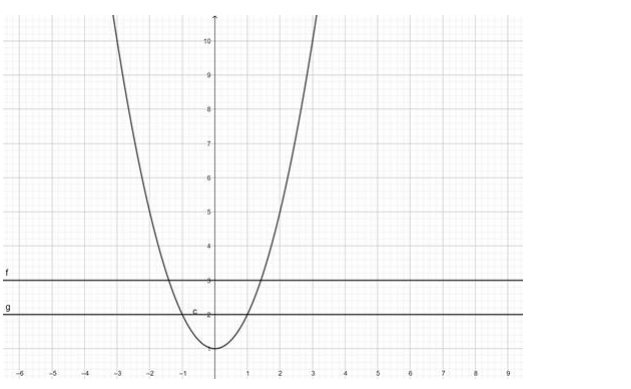# Show that the functionQuestion:

Show that the function $f: R \rightarrow R: f(x)=1+x^{2}$ is many - one into.

Solution:

To show: $f: R \rightarrow R: f(x)=1+x^{2}$ is many - one into.

Proof:

$f(x)=1+x^{2}$

$\Rightarrow y=1+x^{2}$Since the lines cut the curve in 2 equal valued points of $y$ therefore the function $f(x)$ is many one.

The range of $f(x)=[1, \infty) \neq R($ Codomain $)$

$\therefore f(x)$ is not onto

$\Rightarrow f(x)$ is into

Hence, showed that $f: R \rightarrow R: f(x)=1+x^{2}$ is many - one into.